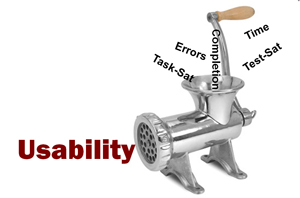# 10 Things to Know about the Single Usability Metric (SUM)

Jeff Sauro, PhDThere is no usability thermometer to tell you how easy to use a website or software application is.

Instead we rely on the outcomes of good and bad experiences which provide evidence for the construct of usability.

Combining multiple usability metrics into a single usability metric (SUM) is something we proposed seven years ago[PDF] and we wrote about in Chapter 9 of Quantifying the User Experience.

Here are 10 things to know about single measures of usability.

1. Usability is the intersection of effectiveness, efficiency and satisfaction (ISO 9241 pt 11). One of the best measures of usability is a combination of metrics that describes each of these aspects.
2. The most common usability metrics are completion rates and errors (effectiveness), task-times (efficiency) and task-level satisfaction (satisfaction). These metrics tend to have a moderate correlation[PDF] with each other of r = .3 to .5. The correlation is strong enough to suggest an overlap (e.g., users that commit more errors tend to take longer) but the correlation isn’t strong enough that one metric can substitute for the other.
3. By averaging together a standardized version of completion rates, task-times, task-level satisfaction and errors you generate a Single Usability Metric (SUM) which summarizes the majority of information in all four measures. By averaging you weight each metric equally. Despite many discussions for determining which metric “counts” more, our analysis found that a simple average is least subjective and reflects the data best (from a principal components analysis[PDF]). Keep in mind that if you weight one metric a lot then you must lessen the weight of another, often to a point where an additional metric does little.
4. You can have 3 metric or 4 metric versions of SUM: Errors are usually the most time consuming and difficult to collect metric (especially in unmoderated testing) so completion rates, task-times and task-satisfaction provide the minimum description of effectiveness, efficiency and satisfaction for a single usability metric.
5. A single usability metric doesn’t replace the individual metrics; it simply summarizes them in a more condensed way like an abstract to a long paper or like the mean summarizes a large set of numbers. With any summarization comes data loss, but the gain in interpretability usually far outweighs the loss—especially considering you don’t “lose” anything as you can always dive into the individual metrics (like you can read the details of a paper).
6. There are a number of reasonable ways to combine usability metrics. One of the best ways we’ve found is to convert everything into a percentage. For discrete metrics (completion rates and errors) this is done by generating a proportion and for continuous metrics (time and satisfaction) we generate a normalized “z-score” and convert it to percentage then average the metrics together.
7. To convert discrete data so they are amenable to combining:
• For completion rates:  they are already in the percentage form.  An 80% completion rate stays as 80%.
• For errors: you need to convert the raw number of errors into a proportion by identifying the opportunities for errors and subtracting this proportion by 1 (so higher proportions are better). If 10 users commit 20 errors and there are 5 opportunities for an error per task the error rate is 20/50 = .40. Subtracting this value from 1 reverses the error rate so higher percentages are better 1-.4 = 60%.
8. To convert continuous data so they are amenable to combining:
• For task-level satisfaction:  if you are using the standardized task-level metric like the SEQ you can use the percentile rank. If you have a 5-point or 7-point scale then common specification limits are 4 (for a 5 point scale) and 5 (for a 7 point scale).  For example, an average score of a 5.6 on a 7 point scale with a standard deviation of 2 becomes (5.6-5)/2 = .3. The .3 is a z-score and gets converted into a percentage =61.7%.
• For task times you need to identify how long a task should take (a specification limit) and subtract the mean time from the successful task attempts. There’s an art to determining[pdf] how long a task should take. For example, if the average time is 50 seconds with a standard deviation of 40 seconds and the spec time is 80 seconds we get a z-score of (50-80)/40  = -.75. The -.75 is a z-score and we convert it to a percentage (which is the area under the curve up to -.75 standard deviations) and we get .2266. We subtract this value from 1 (because we want times to be less than the spec limit) which generates a percentage of 1-.2266 = 77.3%
9. A single usability metric is ideal for dashboards, for comparing competing products[pdf] and tasks when you need a single dependent variable to describe the complex construct of usability. Given the four example metrics shown above, we get a SUM of (80%+60%+61.7%+77.3%)/4 = 69.75%.
10. You can convert raw usability metrics into a SUM score by using the free downloadable Excel spreadsheet or the usability scorecard application.
0
0